Next: Exercises Up: Relativistic Electron Theory Previous: Fine Structure of Hydrogen

# Positron Theory

We have already mentioned that the Dirac equation admits twice as many solutions as it ought to, half of them belonging to states with negative values for the kinetic energy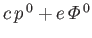. This difficulty was introduced when we passed from Equation (11.16) to Equation (11.18), and is inherent in any relativistic theory.

Let us examine the negative energy solutions of the equation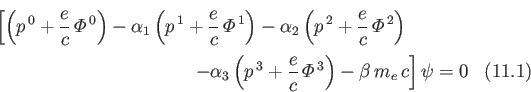a little more closely. For this purpose, it is convenient to use a representation of the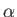's and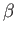in which all the elements of the matrices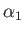,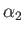, and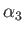are real, and all of those of the matrix representingare imaginary or zero. Such a representation can be obtained from the standard representation by interchanging the expressions forand. If Equation (11.200) is expressed as a matrix equation in this representation, and we then substitute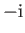for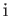, we get [remembering the factorin Equation (11.14)]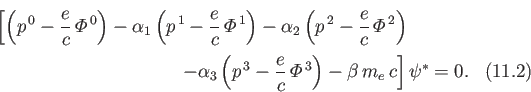Thus, each solution,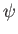, of the wave equation (11.200) has for its complex conjugate,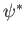, a solution of the wave equation (11.201). Furthermore, if the solution,, of Equation (11.200) belongs to a negative value forthen the corresponding solution,, of Equation (11.201) will belong to a positive value for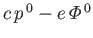. But, the operator in Equation (11.201) is just what we would get if we substituted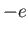for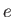in the operator in Equation (11.200). It follows that each negative energy solution of Equation (11.200) is the complex conjugate of a positive energy solution of the wave equation obtained from Equation (11.200) by the substitution offor. The latter solution represents an electron of charge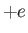(instead of, as we have had up to now) moving through the given electromagnetic field.

We conclude that the negative energy solutions of Equation (11.200) refer to the motion of a new type of particle having the mass of an electron, but the opposite charge . Such particles have been observed experimentally, and are called positrons . Note that we cannot simply assert that the negative energy solutions represent positrons, because this would make the dynamical relations all wrong. For instance, it is certainly not true that a positron has a negative kinetic energy. Instead, we assume that nearly all of the negative energy states are occupied, with one electron in each state, in accordance with the Pauli exclusion principle. An unoccupied negative energy state will now appear as a particle with a positive energy, because to make it disappear we would have to add an electron with a negative energy to the system. We assume that these unoccupied negative energy states correspond to positrons.

The previous assumptions require there to be a distribution of electrons of infinite density everywhere in space. A perfect vacuum is a region of space in which all states of positive energy are unoccupied, and all of those of negative energy are occupied. In such a vacuum, the Maxwell equation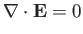(11.200)

must be valid. This implies that the infinite distribution of negative energy electrons does not contribute to the electric field. Thus, only departures from the vacuum distribution contribute to the electric charge density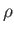in the Maxwell equation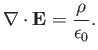(11.201)

In other words, there is a contributionfor each occupied state of positive energy, and a contributionfor each unoccupied state of negative energy.

The exclusion principle ordinarily prevents a positive energy electron from making transitions to states of negative energy. However, it is still possible for such an electron to drop into an unoccupied state of negative energy. In this case, we would observe an electron and a positron simultaneously disappearing, their energy being emitted in the form of radiation. The converse process would consist in the creation of an electron-positron pair from electromagnetic radiation.Next: Exercises Up: Relativistic Electron Theory Previous: Fine Structure of Hydrogen
Richard Fitzpatrick 2016-01-22